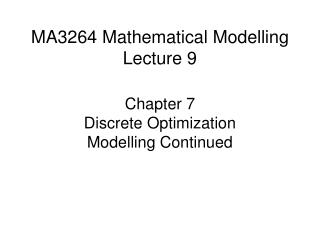DownloadDownload PresentationMA3264 Mathematical Modelling Lecture 9

# MA3264 Mathematical Modelling Lecture 9

Download Presentation## MA3264 Mathematical Modelling Lecture 9

- - - - - - - - - - - - - - - - - - - - - - - - - - - E N D - - - - - - - - - - - - - - - - - - - - - - - - - - -
##### Presentation Transcript

1. MA3264 Mathematical ModellingLecture 9 Chapter 7 Discrete Optimization Modelling Continued

2. Theorem 1 p. 257-258 Level curves Increasing directionof objective function feasible region is blue Question Where is objective function max?

3. Theorem 1 p. 257-258 Increasing directionof objective function Question Where is objective function max?

4. Theorem 1 p. 257-258 Increasing directionof objective function Question Where is objective function max?

5. Example 1 page 261 This Carpenter Problem has different parameters from that on page 238 How many tables and how many bookcases should a carpenter make each week to maximize profit? He realizes a profit of \$25 per table and \$30 per bookcase. He has 690 feet of lumber per week and 120 hours of labor per week. Each table requires 20 feet of lumber and 5 hours of labor. Each bookcase requires 30 feet of lumber and 4 hours of labor.

6. Mathematical Formulation decision variables Maximize objective function Subject to explicitly stated constraints common sense

7. Figure 7.12 p. 261 Question Is the feasible region convex?

8. Slack Variables Question SV satisfy which inequalities?

9. Reformulation Maximize Subject to Question SV satisfy which inequalities?

10. Simplex Method Always assume constraints But manipulate these Remark At each extreme point at least 2 of these 4 variables equal 0.

11. Simplex Method Start at Move to Move to These pairs of independent variables depend on the extreme point At each step 1 independent variable and 1 dependent variable change places

12. Simplex Method Start at Move to Move to In step 1 independent variable becomes dependent since it has the largest negative coeff. in equation for the objective variable

13. Simplex Method Start at Move to Move to The variable becomes independent since the eqn. it is in restricts to be smallest.

14. Simplex Method Start at Move to Move to Equations express dependent as functions of  independent at

15. Simplex Method Start at Move to Move to We pivot to express dependent as functions of  independent at

16. Simplex Method Start at Move to Move to In step 2 independent variable becomes dependent since it has the largest negative coeff. in equation for the objective variable

17. Simplex Method Start at Move to Move to The variable becomes independent since the eqn. it is in restricts to be smallest.

18. Simplex Method Start at Move to Move to We pivot to express dependent as functions of  independent at

19. Simplex Method Start at Move to Move to We stop since in the equation for objective variable all coeff. are nonnegative.

20. Tableau Format Study Example 1 on pages 268-271 Observe that Tableau 0 represents the 3 equations in vufoil 13 Tableau 1 represents the 3 equations in vufoil 15 Tableau 2 represents the 3 equations vufoil in 18

21. Suggested Reading Linear Programming 2: Algebraic Solutions p. 259-263 Linear Programming 3: The Simplex Method p. 263-273

22. Tutorial 9 Due Week 27–31 Oct Problem 1. Modify the Bus Stop Waiting program to write a program to compute the waiting time histogram assuming that people form a queue so that people who arrive first are the first to board a bus. Then RUN this program and compare the waiting times with those without queuing. Problem 2. Do problem 5 on page 259. Suggestion: study how to formulate a Chebyshev approximation problem into a linear program on pages 107-109.

23. Homework 3 Due Friday 31 October 7.4 Project 1 Write a computer program to perform the basic simplex algorithm. The program should print out the sequence of all tableaus from the initial to the final one. It should print out the coordinates of all of the extreme points and the values of the objective function at these points. Then use the program to solve problem 3 on p. 258.